1594366200

# Recurrent Neural Networks for Multilabel Text Classification Tasks

The purpose of this project is to build and evaluate Recurrent Neural Networks(RNNs) for sentence-level classification tasks. I evaluate three architectures: a two-layer Long Short-Term Memory Network(LSTM), a two-layer Bidirectional Long Short-Term Memory Network(BiLSTM), and a two-layer BiLSTM with a word-level attention layer. Although they do learn useful vector representation, BiLSTM with attention mechanism focuses on necessary tokens when learning text representation. To that end, I’m using the 2019 Google Jigsaw published dataset on Kaggle labeled “Jigsaw Unintended Bias in Toxicity Classification.” The dataset includes 1,804,874 user comments, with the toxicity level being between 0 and 1. The final models can be used for filtering online posts and comments, social media policing, and user education.

## Recurrent Neural Networks Overview

RNNs are neural networks used for problems that require sequential data processing. For instance:

• In a sentiment analysis task, a text’s sentiment can be inferred from a sequence of words or characters.
• In a stock prediction task, current stock prices can be inferred from a sequence of past stock prices.

At each time step of the input sequence, RNNs compute the output yt and an internal state update ht using the input xt and the previous hidden-state ht-1. They then pass information about the current time step of the network to the next. The hidden-state ht summarizes the task-relevant aspect of the past sequence of the input up to t, allowing for information to persist over time.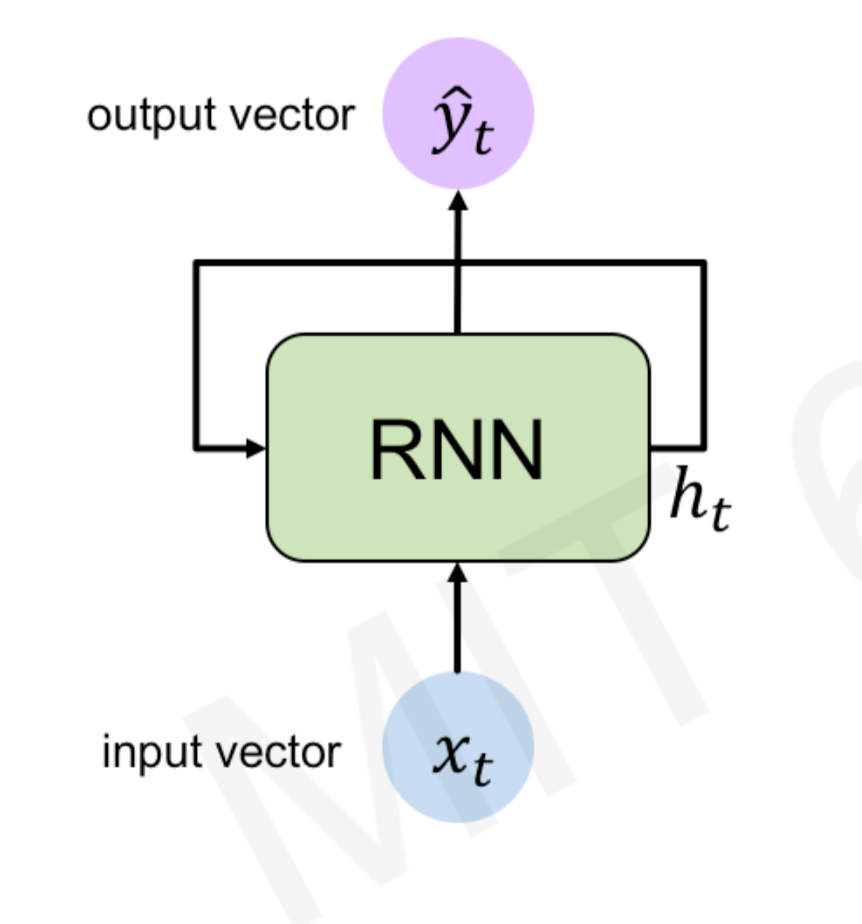Recurrent Neural Network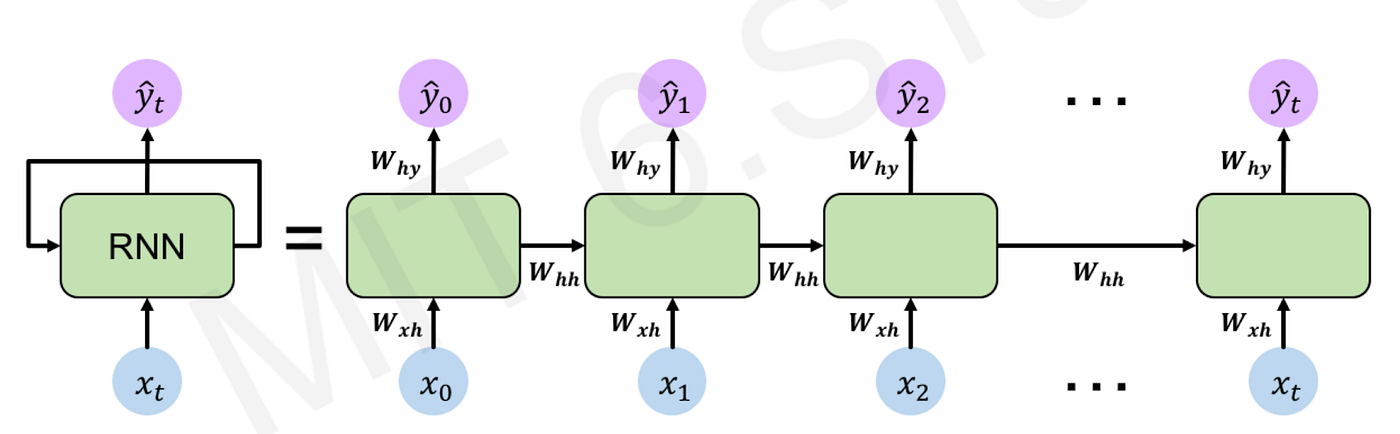Recurrent Neural Network

During training, RNNs re-use the same weight matrices at each time step. Parameter sharing enables the network to generalize to different sequence lengths. The total loss is a sum of all losses at each time step, the gradients with respect to the weights are the sum of the gradients at each time step, and the parameters are updated to minimize the loss function.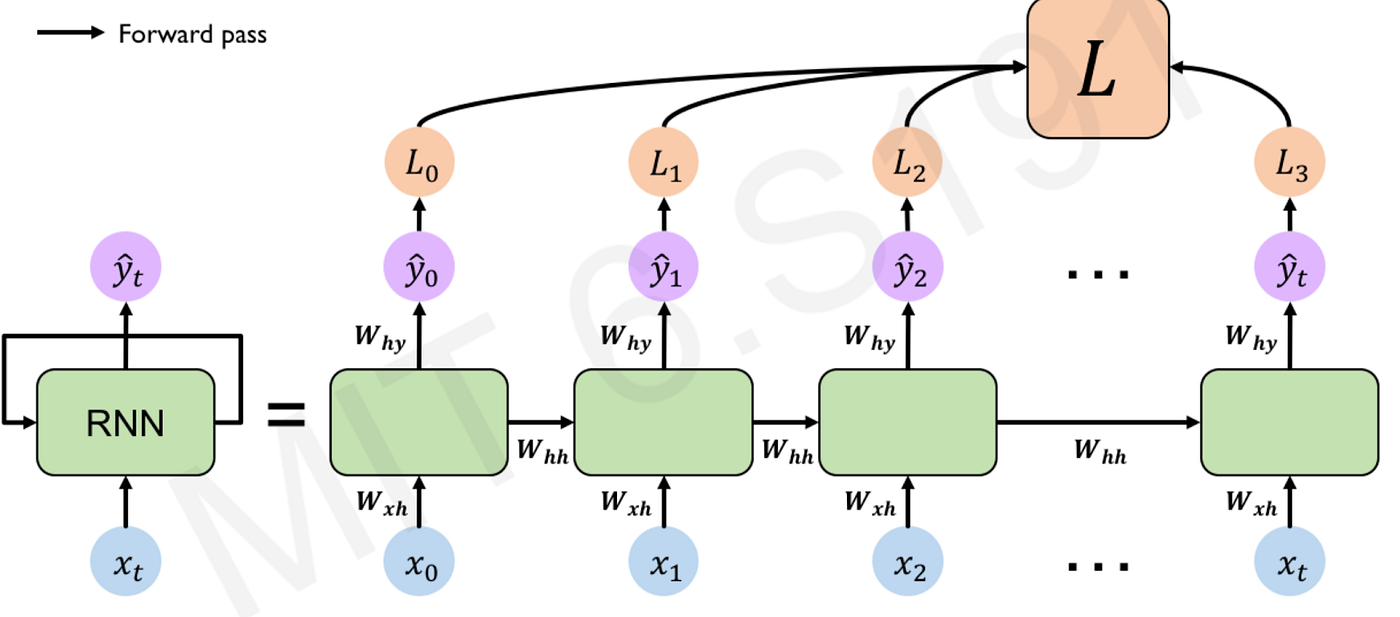forward pass: compute the loss function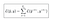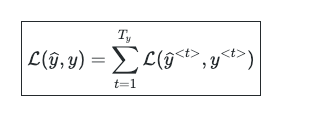loss function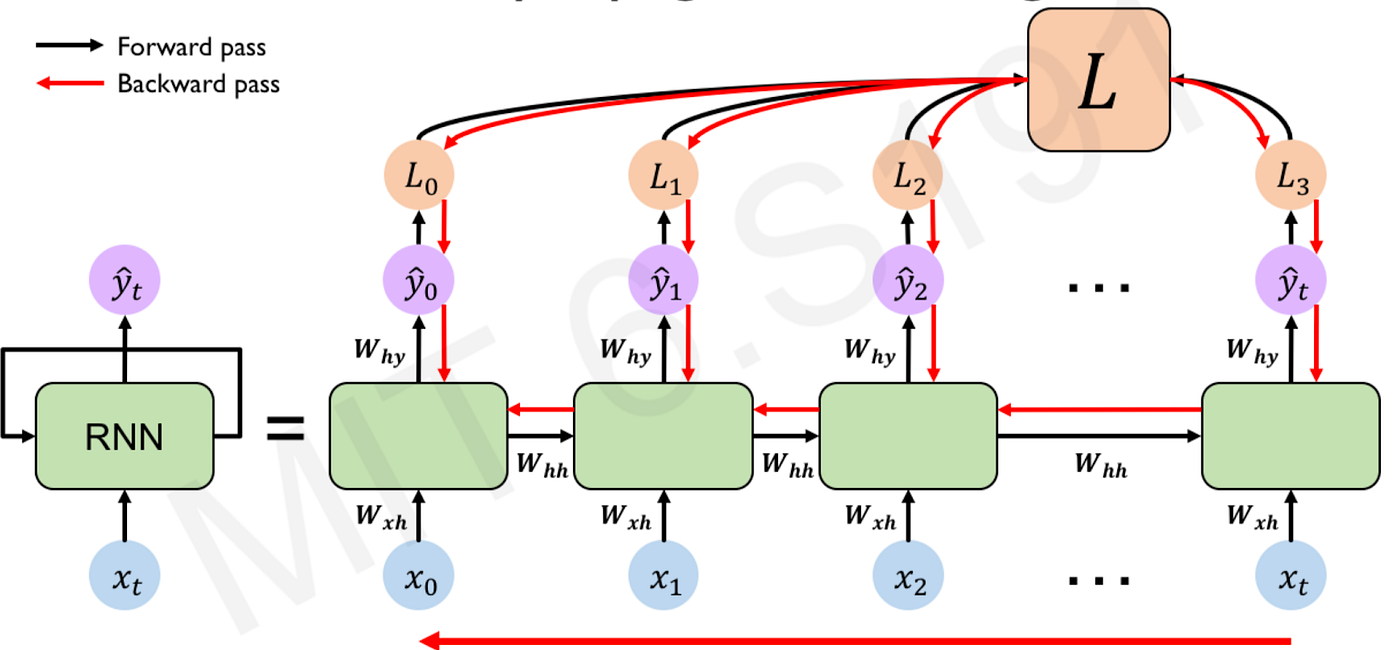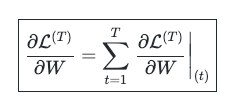Although RNNs learn contextual representations of sequential data, they suffer from the exploding and vanishing gradient phenomena in long sequences. These problems occur due to the multiplicative gradient that can exponentially increase or decrease through time. RNNs commonly use three activation functions: RELU, Tanh, and Sigmoid. Because the gradient calculation also involves the gradient with respect to the non-linear activations, architectures that use a RELU activation can suffer from the exploding gradient problem. Architectures that use Tanh/Sigmoid can suffer from the vanishing gradient problem. Gradient clipping — limiting the gradient within a specific range — can be used to remedy the exploding gradient. However, for the vanishing gradient problem, a more complex recurrent unit with gates such as Gated Recurrent Unit (GRU) or Long Short-Term Memory (LSTM) can be used.

#ai #recurrent-neural-network #attention-network #machine-learning #neural-network

## Buddha Community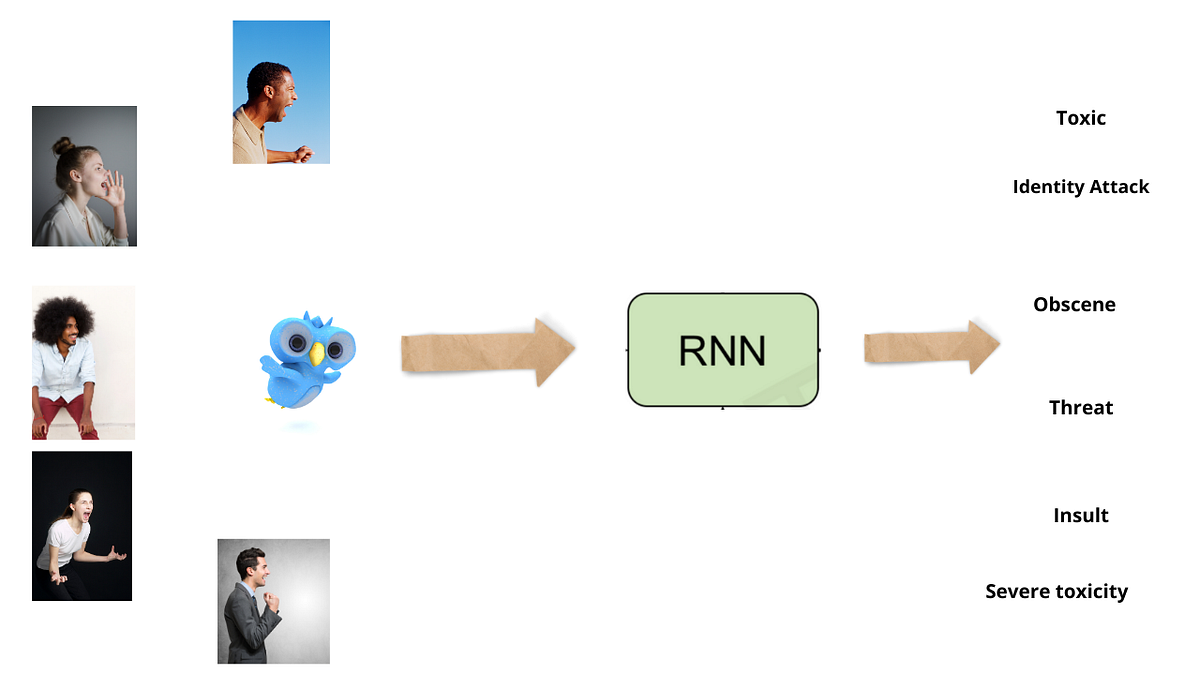1594366200

## Recurrent Neural Networks for Multilabel Text Classification Tasks

The purpose of this project is to build and evaluate Recurrent Neural Networks(RNNs) for sentence-level classification tasks. I evaluate three architectures: a two-layer Long Short-Term Memory Network(LSTM), a two-layer Bidirectional Long Short-Term Memory Network(BiLSTM), and a two-layer BiLSTM with a word-level attention layer. Although they do learn useful vector representation, BiLSTM with attention mechanism focuses on necessary tokens when learning text representation. To that end, I’m using the 2019 Google Jigsaw published dataset on Kaggle labeled “Jigsaw Unintended Bias in Toxicity Classification.” The dataset includes 1,804,874 user comments, with the toxicity level being between 0 and 1. The final models can be used for filtering online posts and comments, social media policing, and user education.

## Recurrent Neural Networks Overview

RNNs are neural networks used for problems that require sequential data processing. For instance:

• In a sentiment analysis task, a text’s sentiment can be inferred from a sequence of words or characters.
• In a stock prediction task, current stock prices can be inferred from a sequence of past stock prices.

At each time step of the input sequence, RNNs compute the output yt and an internal state update ht using the input xt and the previous hidden-state ht-1. They then pass information about the current time step of the network to the next. The hidden-state ht summarizes the task-relevant aspect of the past sequence of the input up to t, allowing for information to persist over time.Recurrent Neural NetworkRecurrent Neural Network

During training, RNNs re-use the same weight matrices at each time step. Parameter sharing enables the network to generalize to different sequence lengths. The total loss is a sum of all losses at each time step, the gradients with respect to the weights are the sum of the gradients at each time step, and the parameters are updated to minimize the loss function.forward pass: compute the loss functionloss functionAlthough RNNs learn contextual representations of sequential data, they suffer from the exploding and vanishing gradient phenomena in long sequences. These problems occur due to the multiplicative gradient that can exponentially increase or decrease through time. RNNs commonly use three activation functions: RELU, Tanh, and Sigmoid. Because the gradient calculation also involves the gradient with respect to the non-linear activations, architectures that use a RELU activation can suffer from the exploding gradient problem. Architectures that use Tanh/Sigmoid can suffer from the vanishing gradient problem. Gradient clipping — limiting the gradient within a specific range — can be used to remedy the exploding gradient. However, for the vanishing gradient problem, a more complex recurrent unit with gates such as Gated Recurrent Unit (GRU) or Long Short-Term Memory (LSTM) can be used.

#ai #recurrent-neural-network #attention-network #machine-learning #neural-network1596825840

## A Comparative Analysis of Recurrent Neural Networks

Recurrent neural networks, also known as RNNs, are a class of neural networks that allow previous outputs to be used as inputs while having hidden states. RNN models are mostly used in the fields of natural language processing and speech recognition.

The vanishing and exploding gradient phenomena are often encountered in the context of RNNs. The reason why they happen is that it is difficult to capture long term dependencies because of multiplicative gradient that can be exponentially decreasing/increasing with respect to the number of layers.

Gated Recurrent Unit (GRU) and Long Short-Term Memory units (LSTM) deal with the vanishing gradient problem encountered by traditional RNNs, with LSTM being a generalization of GRU.

1D Convolution_ layer_ creates a convolution kernel that is convolved with the layer input over a single spatial (or temporal) dimension to produce a tensor of outputs. It is very effective for deriving features from a fixed-length segment of the overall dataset. A 1D CNN works well for natural language processing (NLP).

## DATASET: IMDb Movie Review

TensorFlow Datasets is a collection of datasets ready to use, with TensorFlow or other Python ML frameworks, such as Jax. All datasets are exposed as `[_tf.data.Datasets_](https://www.tensorflow.org/api_docs/python/tf/data/Dataset)`, enabling easy-to-use and high-performance input pipelines.

“imdb_reviews”

This is a dataset for binary sentiment classification containing substantially more data than previous benchmark datasets. It provides a set of 25,000 highly polar movie reviews for training, and 25,000 for testing.

## Import Libraries

``````import pandas as pd
import numpy as np
import matplotlib.pyplot as plt
import seaborn as sns
%matplotlib inline
``````

``````import tensorflow as tf
import tensorflow_datasets

imdb
``````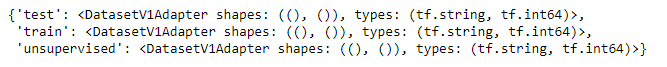``````info
``````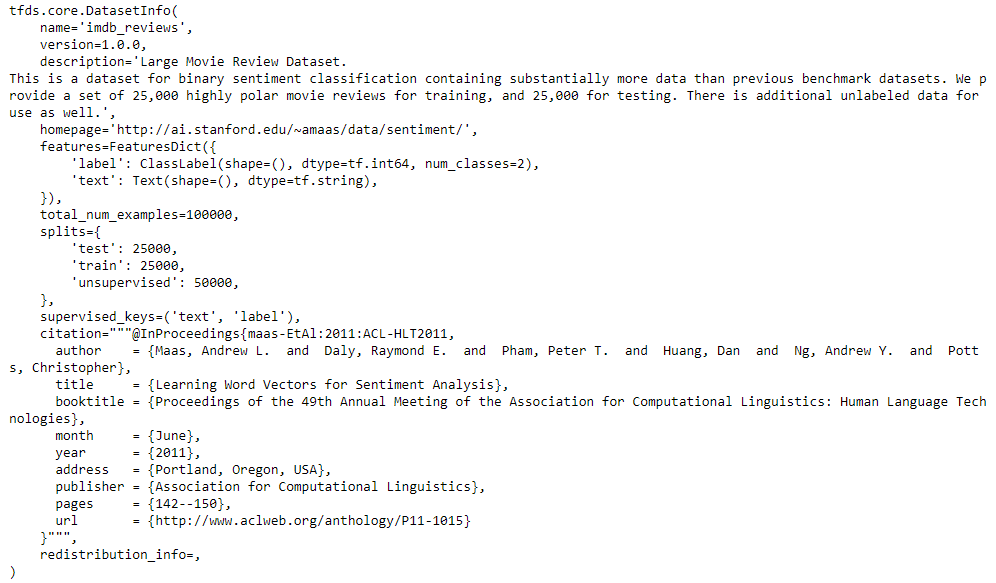## Training and Testing Data

``````train_data, test_data=imdb['train'], imdb['test']

training_sentences=[]
training_label=[]
testing_sentences=[]
testing_label=[]
for s,l in train_data:
training_sentences.append(str(s.numpy()))
training_label.append(l.numpy())
for s,l in test_data:
testing_sentences.append(str(s.numpy()))
testing_label.append(l.numpy())
training_label_final=np.array(training_label)
testing_label_final=np.array(testing_label)
``````

``````vocab_size=10000
embedding_dim=16
max_length=120
trunc_type='post'
oov_tok='<oov>'
from tensorflow.keras.preprocessing.text import Tokenizer
tokenizer= Tokenizer(num_words=vocab_size, oov_token=oov_tok)
tokenizer.fit_on_texts(training_sentences)
word_index=tokenizer.word_index
sequences=tokenizer.texts_to_sequences(training_sentences)
testing_sequences=tokenizer.texts_to_sequences(testing_sentences)
from tensorflow.keras.models import Sequential
from tensorflow.keras.layers import Dense, Dropout, Embedding
``````

## Multi-layer Bidirectional LSTM

#imdb #convolutional-network #long-short-term-memory #recurrent-neural-network #gated-recurrent-unit #neural networks1650870267

## Navigating Between DOM Nodes in JavaScript

In the previous chapters you've learnt how to select individual elements on a web page. But there are many occasions where you need to access a child, parent or ancestor element. See the JavaScript DOM nodes chapter to understand the logical relationships between the nodes in a DOM tree.

DOM node provides several properties and methods that allow you to navigate or traverse through the tree structure of the DOM and make changes very easily. In the following section we will learn how to navigate up, down, and sideways in the DOM tree using JavaScript.

## Accessing the Child Nodes

You can use the `firstChild` and `lastChild` properties of the DOM node to access the first and last direct child node of a node, respectively. If the node doesn't have any child element, it returns `null`.

#### Example

``````<div id="main">
<p id="hint"><span>This is some text.</span></p>
</div>

<script>
var main = document.getElementById("main");
console.log(main.firstChild.nodeName); // Prints: #text

var hint = document.getElementById("hint");
console.log(hint.firstChild.nodeName); // Prints: SPAN
</script>``````

Note: The `nodeName` is a read-only property that returns the name of the current node as a string. For example, it returns the tag name for element node, `#text` for text node, `#comment` for comment node, `#document` for document node, and so on.

If you notice the above example, the `nodeName` of the first-child node of the main DIV element returns #text instead of H1. Because, whitespace such as spaces, tabs, newlines, etc. are valid characters and they form #text nodes and become a part of the DOM tree. Therefore, since the `<div>` tag contains a newline before the `<h1>` tag, so it will create a #text node.

To avoid the issue with `firstChild` and `lastChild` returning #text or #comment nodes, you could alternatively use the `firstElementChild` and `lastElementChild` properties to return only the first and last element node, respectively. But, it will not work in IE 9 and earlier.

#### Example

``````<div id="main">
<p id="hint"><span>This is some text.</span></p>
</div>

<script>
var main = document.getElementById("main");
main.firstElementChild.style.color = "red";

var hint = document.getElementById("hint");
hint.firstElementChild.style.color = "blue";
</script>``````

Similarly, you can use the `childNodes` property to access all child nodes of a given element, where the first child node is assigned index 0. Here's an example:

#### Example

``````<div id="main">
<p id="hint"><span>This is some text.</span></p>
</div>

<script>
var main = document.getElementById("main");

// First check that the element has child nodes
if(main.hasChildNodes()) {
var nodes = main.childNodes;

// Loop through node list and display node name
for(var i = 0; i < nodes.length; i++) {
}
}
</script>``````

The `childNodes` returns all child nodes, including non-element nodes like text and comment nodes. To get a collection of only elements, use `children` property instead.

#### Example

``````<div id="main">
<p id="hint"><span>This is some text.</span></p>
</div>

<script>
var main = document.getElementById("main");

// First check that the element has child nodes
if(main.hasChildNodes()) {
var nodes = main.children;

// Loop through node list and display node name
for(var i = 0; i < nodes.length; i++) {
}
}
</script>``````1594524540

## The Recurrent Neural Network (RNNs)

A recurrent neural network (RNN) is an input node (hidden layer) that feeds sigmoid activation. The way an RNN does this is to take the output of one neuron and return it as input to another neuron or feed the input of the current time step to the output of earlier time steps. Here you feed the input from the previous times step by step into the input of the current times and vice versa.This can be used in a variety of ways, such as through learning gates with known variations or a combination of sigmoid activation and a number of other types of neural networks.

Some of the applications for RNNs include predicting energy demand, predicting stock prices, and predicting human behavior. RNNs are modeled over time — based and sequence-based data, but they are also useful in a variety of other applications.

A recurrent neural network is an artificial neural network used for deep learning, machine learning, and other forms of artificial intelligence (AI). They have a number of attributes that make them useful for tasks where data needs to be processed sequentially.

To get a little more technical, recurring neural networks are designed to learn a sequence of data by traversing a hidden state from one step of the sequence to the next, combined with the input, and routing it back and forth between the inputs. RNN are neural networks that are designed for the effective handling of sequential data but are also useful for non-sequential data.

These types of data include text documents that can be seen as a sequence of words or audio files in which you can see a sequence of sound frequencies and times. The more information about the output layer is available, the faster it can be read and sequenced, and the better its performance.

#recurrent-neural-network #lstm #rnn #artificial-intelligence #neural network1623135499

## No Code introduction to Neural Networks

### The simple architecture explained

Neural networks have been around for a long time, being developed in the 1960s as a way to simulate neural activity for the development of artificial intelligence systems. However, since then they have developed into a useful analytical tool often used in replace of, or in conjunction with, standard statistical models such as regression or classification as they can be used to predict or more a specific output. The main difference, and advantage, in this regard is that neural networks make no initial assumptions as to the form of the relationship or distribution that underlies the data, meaning they can be more flexible and capture non-standard and non-linear relationships between input and output variables, making them incredibly valuable in todays data rich environment.

In this sense, their use has took over the past decade or so, with the fall in costs and increase in ability of general computing power, the rise of large datasets allowing these models to be trained, and the development of frameworks such as TensforFlow and Keras that have allowed people with sufficient hardware (in some cases this is no longer even an requirement through cloud computing), the correct data and an understanding of a given coding language to implement them. This article therefore seeks to be provide a no code introduction to their architecture and how they work so that their implementation and benefits can be better understood.

Firstly, the way these models work is that there is an input layer, one or more hidden layers and an output layer, each of which are connected by layers of synaptic weights¹. The input layer (X) is used to take in scaled values of the input, usually within a standardised range of 0–1. The hidden layers (Z) are then used to define the relationship between the input and output using weights and activation functions. The output layer (Y) then transforms the results from the hidden layers into the predicted values, often also scaled to be within 0–1. The synaptic weights (W) connecting these layers are used in model training to determine the weights assigned to each input and prediction in order to get the best model fit. Visually, this is represented as:

#machine-learning #python #neural-networks #tensorflow #neural-network-algorithm #no code introduction to neural networks